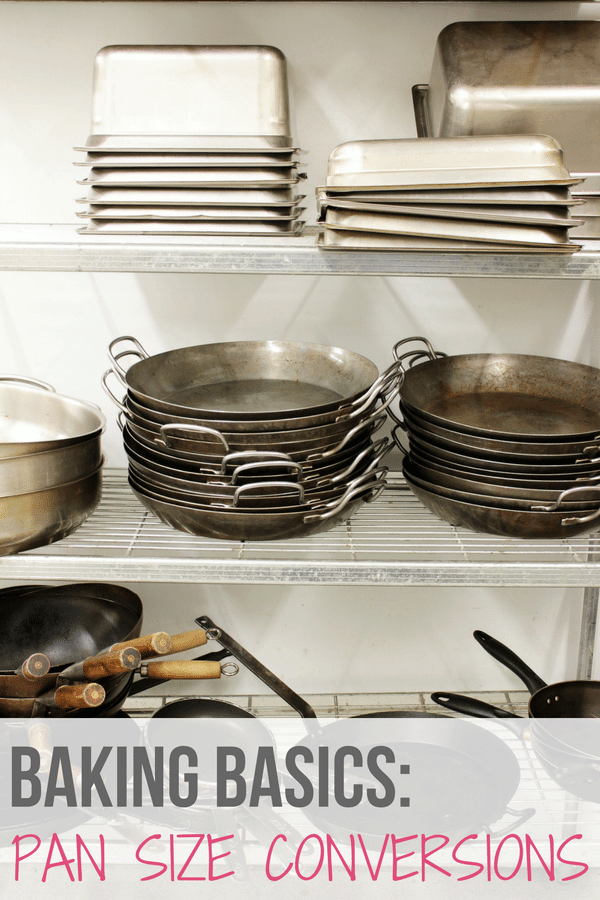# Baking Basics: Converting Pan SizesHappy Tuesday and welcome to another Baking Basics installment!

One of the most common questions that I receive is how to adapt or scale a recipe if you need to use a different size pan. Will there be too much batter, should it be baked longer or shorter, etc.? While your first inclination might just be to take the surface measurements of the pan (8-inch, 9-inch, 9×13-inch, etc.), the more important measurement is VOLUME. This gives you a much better indication of how much batter the pan can hold and if you will need to do any scaling or time adjustments.

Below is a list of common pan sizes and volumes, along with a link to more non-traditional pan sizes!

### PAN SIZE CONVERSIONS

(Dimension) 1 inch = 2.54cm  (Volume) 1 cup = 237 mL

Round Pans:
6 x 2 inches = 15 x 5 cm = 4 cups = 948ml
8 x 2 inches = 20 x 5 cm = 6 cups = 1.4 liters
9 x 2 inches = 23 x 5 cm = 8 cups = 1.9 liters

Springform Pans:
9 x 2½ inches = 23 x 6 cm = 10 cups = 2.4 liters
9 x 3 inches  = 23 x 8 cm = 12 cups = 2.8 liters
10 x 2½ inches = 25 x 6 cm = 12 cups = 2.8 liters

Bundt Pans:
7½ x 3 inches = 19 x 8 cm = 6 cups = 1.4 liters
9 x 3 inches = 23 x 8 cm = 9 cups = 2.1 liters
10 x 3½ inches = 25 x 9 cm = 12 cups = 2.8 liters

Tube Pans:
8 x 3 inches = 20 x 8 cm = 9 cups = 2.1 liters
9 x 3 inches = 23 x 8 cm =  12 cups = 2.8 liters
10 x 4 inches = 25 x 10 cm = 16 cups = 3.8 liters

Square Pans:
8 x 8 x 2 inches = 20 x 20 x 5 cm = 8 cups = 1.9 liters
9 x 9 x 2 inches = 23 x 23 x 5 cm = 10 cups = 2.4 liters
10 x 10 x 2 inches = 25 x 25 x 5 cm = 12 cups = 2.8 liters

Rectangular Pans:
11 x 7 x 2 inches = 28 x 18 x 5 cm = 6 cups = 1.4 liters
13 x 9 x 2 inches = 33 x 23 x 5 cm = 14 cups = 3.3 liters

Jelly Roll Pans:
10½ x 15½ x 1 = 27 x 39 x 2.5 cm = 10 cups = 2.4 liters
12½ x 17½ x 1 = 32 x 44 x 2.5 cm = 12 cups = 2.8 liters

Loaf Pans:
8 x 4 x 2½ inches = 20 x 10 x 6 cm = 4 cups = 948 ml
8½ x 4½ x 2½ inches = 21 x 11 x 6 cm = 6 cups = 1.4 liters
9 x 5 x 3 inches = 23 x 13 x 8 cm = 8 cups = 1.9 liters

Additional Resource: You can find even more obscure pan sizes with measurements at Joyofbaking.com.

### BAKE ON!

5 Mistakes to Avoid When Preparing a Recipe

High Altitude Baking Tips

Flour 101: The Definitive Guide to the Different Types of Baking Flours

Cocoa Powder 101: Natural Unsweetened vs Dutch-Process

Baking Soda vs. Baking Powder: Everything You Need to Know!

Why You Should ALWAYS Weigh Your Ingredients

Chocolate 101

A List of My Must-Have Ingredients

My Essential Baking Equipment